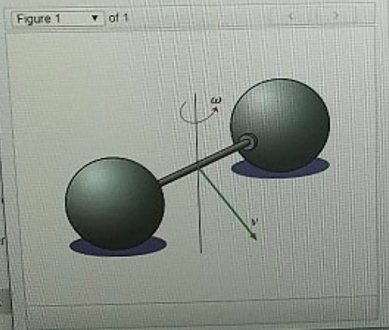# Problem: This problem illustrates the two contributions to the kinetic energy of an extended object: rotational kinetic energy and translational kinetic energy. You are to find the total kinetic energy Ktotal of a dumbbell of mass m when it is rotating with angular speed v (Figure 1) Denote the dumbbell’s moment of inertia about its center of mass by Icm. Note that if you approximate the spheres as point masses of mass m/2 each located a distance r from the center and ignore the moment of inertia of the connecting rod, then the moment of inertia of the dumbbell is given by Icm = mr2 but this fact will not be necessary for this problemFind the total kinetic energy Ktot of the dumbbell.Express your answer in terms of m, v, Icm and w.

###### FREE Expert Solution

Total kinetic energy:

$\overline{){{\mathbf{K}}}_{\mathbf{t}\mathbf{o}\mathbf{t}}{\mathbf{=}}{{\mathbf{K}}}_{\mathbf{t}\mathbf{r}\mathbf{a}\mathbf{n}\mathbf{s}\mathbf{l}\mathbf{a}\mathbf{t}\mathbf{i}\mathbf{o}\mathbf{n}\mathbf{a}\mathbf{l}}{\mathbf{+}}{{\mathbf{K}}}_{\mathbf{r}\mathbf{o}\mathbf{t}\mathbf{a}\mathbf{t}\mathbf{i}\mathbf{o}\mathbf{n}\mathbf{a}\mathbf{l}}}$

Translational kinetic energy:

$\overline{){{\mathbf{K}}}_{\mathbf{t}\mathbf{r}\mathbf{a}\mathbf{n}\mathbf{s}\mathbf{l}\mathbf{a}\mathbf{t}\mathbf{i}\mathbf{o}\mathbf{n}\mathbf{a}\mathbf{l}}{\mathbf{=}}\frac{\mathbf{1}}{\mathbf{2}}{{\mathbf{m}}}_{\mathbf{t}\mathbf{o}\mathbf{t}\mathbf{a}\mathbf{l}}{{{\mathbf{v}}}_{\mathbf{c}\mathbf{m}}}^{{\mathbf{2}}}}$

93% (348 ratings)###### Problem Details

This problem illustrates the two contributions to the kinetic energy of an extended object: rotational kinetic energy and translational kinetic energy. You are to find the total kinetic energy Ktotal of a dumbbell of mass m when it is rotating with angular speed v (Figure 1) Denote the dumbbell’s moment of inertia about its center of mass by Icm. Note that if you approximate the spheres as point masses of mass m/2 each located a distance r from the center and ignore the moment of inertia of the connecting rod, then the moment of inertia of the dumbbell is given by Icm = mr2 but this fact will not be necessary for this problemFind the total kinetic energy Ktot of the dumbbell.
Express your answer in terms of m, v, Icm and w.

Frequently Asked Questions

What scientific concept do you need to know in order to solve this problem?

Our tutors have indicated that to solve this problem you will need to apply the Intro to Rotational Kinetic Energy concept. You can view video lessons to learn Intro to Rotational Kinetic Energy. Or if you need more Intro to Rotational Kinetic Energy practice, you can also practice Intro to Rotational Kinetic Energy practice problems.

What professor is this problem relevant for?

Based on our data, we think this problem is relevant for Professor Tsybychev's class at SUNY.